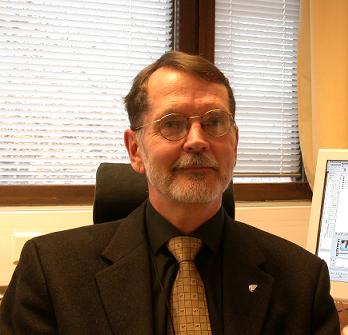Enter the content which will be displayed in sticky bar

# MemberDr. Ari Lehto
local time: 2023-10-01 08:38 (+02:00 )
Dr. Ari Lehto (Abstracts)
Titles
• [Updated 6 years ago]
• [Updated 6 years ago]
• [Updated 6 years ago]
Abstracts Details
• [Updated 6 years ago]

Nonlinear Dynamics, V55, N3, pp. 279-298 (Feb 2009).  Invariant and long-lived physical properties and structures of matter are modeled by intrinsic rotations in three and four degrees of freedom. The rotations are quantized starting from the Planck scale by using a nonlinear 1/r potential and period doubling?a common property of nonlinear dynamical systems. The absolute values given by the scale-independent model fit closely with observations in a wide range of scales. A comparison is made between the values calculated from the model and the properties of the basic elementary particles, particle processes, planetary systems, and other physical phenomena. The model also shows that the perceived forces can be divided into two categories: (1) force is always attractive, like in gravitation and (2) force is attractive or repulsive, like in electrostatics.

• [Updated 6 years ago]

Invariant properties and structures of matter are modeled by internal
period-like degrees of freedom. Invariance then means periods, which
remain unaltered over time. Period doubling is a phenomenon common to
nonlinear dynamical systems. In this model the doubling process is
generalized into multiple dimensions and utilized to bring about
sub-harmonic frequencies, which generate decreasing energies and
increasing sizes. It is assumed that period doubling takes place at the
Planck scale, and therefore the Planck units are used as reference. The
sub-harmonics can be converted into several other physical quantities
by well known physical relations. A certain class of sub-harmonics is
stable and the elementary electric charge (squared), rest energies and
magnetic moments of the electron-positron and proton-antiproton pairs
are shown to belong to this class. It is suggested that the structure
of the Solar system results from period doubling, too.

• [Updated 6 years ago]

One of the most interesting questions in modern physics is the possible relation of the Planck scale to our perceived world. The Planck energy (1022 MeV) is extremely large as compared to the rest energies of the elementary particles and the Planck length (10-35 m) is too short to be directly connected to any real world distances. Why the Planck scale is interesting is that it is absolute, as it is determined by the natural constants h, c, G and eo. Another reason is that the Planck scale may represent the ultimate "graininess", i.e. the basic structure, of the space-time and matter.

It is well known that nonlinear systems show universal behavior in the form of period doubling, which is the same as frequency and energy halving. If period doubling is applied to the Planck energy Eo= h/to, an absolute and unadjustable set of sublevels is borne. Spatial period doubling will correspondingly yield a set of increasing lengths. The rest energy of the electron-positron pair is given directly by a Planck energy sublevel, whereas the nucleon rest energies originate from a sum energy of two adjacent sublevels.
It is also shown that the value of the elementary electric charge squared, which is proportional to energy, results from the Planck charge squared by the same period doubling process.

It is further shown that the planets in the Solar system occupy orbits, the radii of which can be calculated from the Planck length by spatial period doubling. A spectrum of velocities can be calculated from the speed of light by the same process. These velocities fit the consequent orbital velocities of the planets and the quantized redshifts of galaxies, if redshift is interpreted as velocity.

A hypothesis is made that the invariant properties and structures of matter are related to periodic structures obtained by a period doubling process in three and four dimensional nonlinear systems.

PACS numbers: 04.20.Gz, 05.45.-a, 05.45.Mt